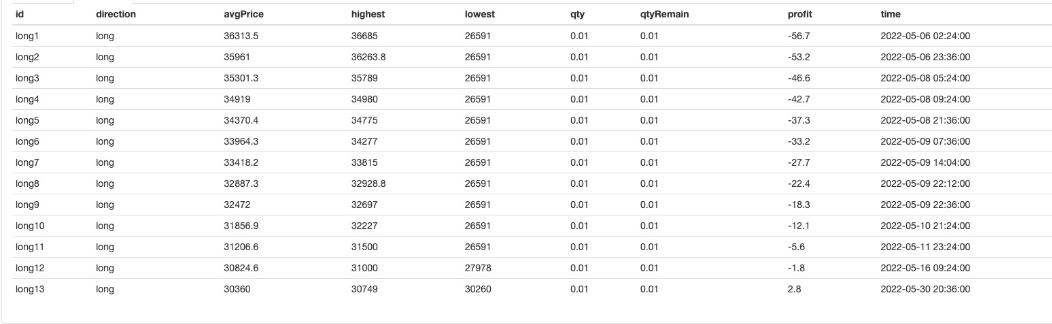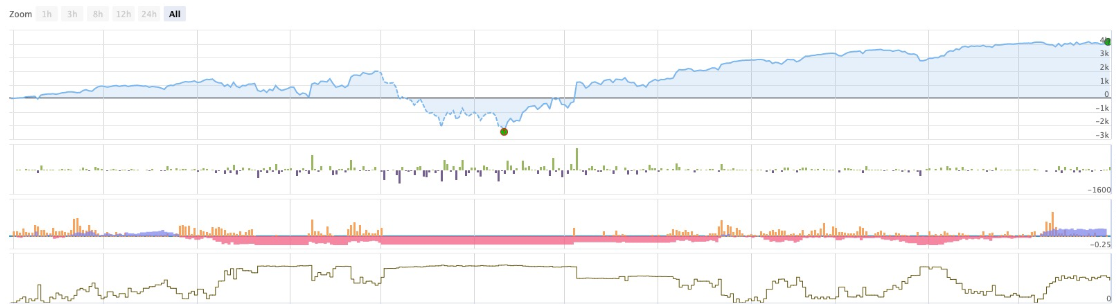# If you don't know how to write a strategy in such an easy-to-learn and easy-to-use Pine language...

Author: Lydia, Created: 2023-02-16 14:55:00, Updated: 2023-09-18 20:18:52## If you don’t know how to write a strategy in such an easy-to-learn and easy-to-use Pine language…

The number of open source strategies on TradingView is large. It is a pity that so many excellent strategies, ideas, and indicators cannot be used in real bot. Seeing this, FMZ, which is committed to popularizing quantitative trading technology to many traders, naturally cannot suppress this urge to solve the problem!

This experience sharing is absolutely to be offered!

So, after trekking through the world of programming and developing code, going through 9*9=81 pits, surviving countless sleepless nights, and piling up a mountain of empty Red Bull cans in the corner. Finally, FMZ supports and is compatible with Pine language, and all kinds of Pine scripts of TradingView can be used.

When it comes to the Pine language, I have only recently taught myself. But to be honest, the Pine language for quantitative trading is really easy to use and easy to learn. What? Do not believe? Let me write a grid strategy for you:

``````/*backtest
start: 2021-06-01 00:00:00
end: 2022-05-23 00:00:00
period: 1h
basePeriod: 1m
exchanges: [{"eid":"Bitfinex","currency":"BTC_USD"}]
args: [["v_input_float_1",500],["v_input_string_1",2],["v_input_float_2",0.01],["v_input_int_1",20],["v_input_int_2",500],["RunMode",1,358374],["MinStock",0.001,358374]]
*/

strategy(overlay=true)

varip beginPrice = 0
var spacing = input.float(-1, title="Spacing prices")
var dir = input.string("long", title="Directions", options = ["long", "short", "both"])
var amount = input.float(-1, title="Order quantity")
var numbers = input.int(-1, title="Number of grids")
var profit = input.int(-1, title="Profit spreads") / syminfo.mintick

if spacing == -1 and amount == -1 and numbers == -1 and profit == -1
runtime.error("Parameter errors")

if not barstate.ishistory and beginPrice == 0
beginPrice := close

ret = "notFound"
for i = 0 to strategy.opentrades - 1
ret

// Real-time K-line stage
if not barstate.ishistory
// Retrieve grid
for i = 1 to numbers
// Going long
direction = dir == "both" ? "long" : dir
plot(beginPrice-i*spacing, direction+str.tostring(i), color.green)
if direction == "long" and beginPrice-i*spacing > 0 and beginPrice-i*spacing < close and findTradeId(direction+str.tostring(i)) == "notFound"
strategy.order(direction+str.tostring(i), strategy.long,  qty=amount, limit=beginPrice-i*spacing)
strategy.exit("exit-"+direction+str.tostring(i), direction+str.tostring(i), qty_percent=100, profit=profit)
// Going short
direction := dir == "both" ? "short" : dir
plot(beginPrice+i*spacing, direction+str.tostring(i), color.red)
if direction == "short" and beginPrice+i*spacing > close and findTradeId(direction+str.tostring(i)) == "notFound"
strategy.order(direction+str.tostring(i), strategy.short, qty=amount, limit=beginPrice+i*spacing)
strategy.exit("exit-"+direction+str.tostring(i), direction+str.tostring(i), qty_percent=100, profit=profit)
``````

FMZ’s real bot, backtesting tools, and numerous functions combined with the simplicity of the Pine language are a great addition! Including the parameter setting and backtesting configuration code, the total code is less than 50 lines. No more headaches to write a grid strategy for beginners.

Of course, this strategy is a grid strategy, which also has flaws, and it is not a money printing machine that always wins. The key depends on the usage and parameters. We will focus more on how to write strategies easily to implement our own trading logic, and make money by writing strategies and trading ourselves. It’s so cool not to ask for help!

### Code explanation

I'll explain to you all, the code is simple and easy to understand, with such an easy to learn and use Pine language, if you still can not write a strategy, then I will… tell you in details!

The content enclosed by `/*backtest` and `*/` at the beginning is the backtest configuration code of FMZ. This is the function of FMZ, not the content of Pine language. Of course, you can leave this part out, and you will click the parameter control manually to set the backtest configuration and parameters during backtesting.

``````/*backtest
start: 2021-06-01 00:00:00
end: 2022-05-23 00:00:00
period: 1h
basePeriod: 1m
exchanges: [{"eid":"Bitfinex","currency":"BTC_USD"}]
args: [["v_input_float_1",500],["v_input_string_1",2],["v_input_float_2",0.01],["v_input_int_1",20],["v_input_int_2",500],["RunMode",1,358374],["MinStock",0.001,358374]]
*/
``````

The next code:

``````strategy(overlay=true)

varip beginPrice = 0
var spacing = input.float(-1, title="Spacing prices")
var dir = input.string("long", title="Directions", options = ["long", "short", "both"])
var amount = input.float(-1, title="Order quantity")
var numbers = input.int(-1, title="Number of grids")
var profit = input.int(-1, title="Profit points") / syminfo.mintick
``````
• `strategy(overlay=true)`: It is used to set some options of the script, overlay=true, which is to assign true value to the parameter `overlay`, so that when drawing the chart, it is drawn on the main chart (K-line chart is the main chart, it can be understood so simply).
• `varip beginPrice = 0`: A variable beginPrice is declared with the keyword varip with an initial value of 0, which is used as the initial price for the grid.
• `var spacing = input.float(-1, title="Spacing prices")`: Set a strategy parameter, the parameter name is “spacing price”, which is the spacing of each grid point, setting 100 means that the price will trade once every 100.
• `var dir = input.string("long", title="Directions", options = ["long", "short", "both"])`: Set a strategy parameter named “direction”, this parameter is a drop-down box with options long, short, and both, which means that the grid is long only, short only, and both, respectively.
• `var amount = input.float(-1, title="Order quantity")`: Set a parameter to control the volume of trades at each grid point trade.
• `var numbers = input.int(-1, title="Number of grids")`: The number of grid points, setting 20 is 20 grid points in one direction.
• `var profit = input.int(-1, title="Profit spreads") / syminfo.mintick`: Set a parameter to control the profit margin of each grid point position before closing the position.

Next, look at the code:

``````if spacing == -1 and amount == -1 and numbers == -1 and profit == -1
runtime.error("Parameter errors")
``````

It means that if any parameters such as spacing, amount, numbers, and profit are not set, the default is -1, and the strategy will stop (you can’t operate blindly without setting parameters)

Go on !

``````if not barstate.ishistory and beginPrice == 0
beginPrice := close
``````

What this means here is that when the strategy is in the real-time K-line stage and beginPrice == 0, change the value of beginPrice to the current latest price. It can be understood that when the strategy is officially running, the initial current price is the initial price of the grid. Because the script has a historical K-line BAR stage, the strategy will execute the logic once in the historical BAR stage, and it is definitely meaningless to arrange the grid on the historical BAR.

What is the historical BAR stage?

To give a simple example, at the current moment A, the strategy starts to run, and the strategy obtains a data with 100 K-line BARs. With time going by, 100 BARs shall become 101, 102…N. When it starts running from the moment A, the 101st BAR is the real-time K-line stage, and this time is the latest real-time data. Then from the 1st BAR to the 100th BAR, these are the historical market prices that have passed, but the strategy will also run on these historical market prices, so this stage is the historical K-line stage.

`barstate.ishistory` this is a built-in variable in the Pine language, `barstate.ishistory` is true if the current BAR is a historical BAR, and false if it is not a historical BAR. So when not barstate.ishistory is true, it is in the real-time K-line stage.

Next, a function is created

``````findTradeId(id) =>
ret = "notFound"
for i = 0 to strategy.opentrades - 1
ret
``````

The role of this function is to find out whether a certain id exists in all orders that have currently opened a position. If there is a findTradeId function call, it will return the ID of the existing order (note that this ID is not the order ID of the exchange, it is the name given to the order by the strategy or understood as a label), if it does not exist, the string “notFound” is returned.

The next step is to start the grid sheet:

``````// Real-time K-line stage
if not barstate.ishistory
// Retrieve grid
for i = 1 to numbers
// Going long
direction = dir == "both" ? "long" : dir
plot(beginPrice-i*spacing, direction+str.tostring(i), color.green)
if direction == "long" and beginPrice-i*spacing > 0 and beginPrice-i*spacing < close and findTradeId(direction+str.tostring(i)) == "notFound"
strategy.order(direction+str.tostring(i), strategy.long,  qty=amount, limit=beginPrice-i*spacing)
strategy.exit("exit-"+direction+str.tostring(i), direction+str.tostring(i), qty_percent=100, profit=profit)
// Going short
direction := dir == "both" ? "short" : dir
plot(beginPrice+i*spacing, direction+str.tostring(i), color.red)
if direction == "short" and beginPrice+i*spacing > close and findTradeId(direction+str.tostring(i)) == "notFound"
strategy.order(direction+str.tostring(i), strategy.short, qty=amount, limit=beginPrice+i*spacing)
strategy.exit("exit-"+direction+str.tostring(i), direction+str.tostring(i), qty_percent=100, profit=profit)
``````

The for loop is used, and the number of loops is determined according to the value of the numbers parameter, that is, the corresponding number of orders are arranged. Set the direction according to the dir parameter. Use the findTradeId function to find out whether the order of the label at the current grid position has been opened, and only place the planned order if there is no open position (if the position is opened, it cannot be repeated). To place an order, use the strategy.order function to specify the limit parameter as a planned order. Place the corresponding closing order while place the planned order. The closing order uses the strategy.exit function, specifies the profit parameter, and specifies the profit points.Looking at the profit curve, we can see that the grid is also risky. It is not a guaranteed win. It is just that the risk of expanding the grid on a large scale is a little smaller.

Well, if you don’t know how to write a strategy in such an easy-to-learn and easy-to-use Pine language, then I…

Related

More